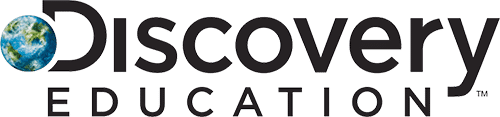## OVERVIEW

Comparable to college and university calculus, this course helps prepare you for the Calculus AB Advanced Placement exam. Study limits, continuity, differentiation, integrated algebraic, trigonometric, and transcendental functions, and the applications of derivatives and integrals.

## MAJOR CONCEPTS

Limits and Continuity

• Using Limits to Analyze Instantaneous Change
• Estimating Limit Values from Graphs and Tables
• Determining Limits Using Algebraic Properties and Manipulation
• Selecting Procedures for Determining Limits
• Squeeze Theorem and Representations of Limits
• Determining Continuity and Exploring Discontinuity
• Connecting Limits, Infinity, and Asymptotes
• The Intermediate Value Theorem (IVT)

Differentiation: Definition and Fundamental Properties

• Average and Instantaneous Rates of Change and the Derivative Definition
• Determining Differentiability and Estimating Derivatives
• Derivative Rules: Constant, Sum, Difference, Constant Multiple, and Power
• The Product Rule and the Quotient Rule
• Derivatives of Trigonometric Functions
• Derivatives of Exponential and Logarithmic Functions

Differentiation: Composite, Implicit, and Inverse Functions

• The Chain Rule
• Implicit Differentiation
• Differentiating Inverse Functions
• Differentiating Inverse Trigonometric Functions
• Selecting Procedures for Calculating Derivatives
• Calculating Higher-Order Derivatives

Contextual Applications of Differentiation

• Interpreting and Applying the Derivative in Motion
• Rates of Change in Applied Contexts Other Than Motion
• Related Rates
• Approximating Values of a Function Using Local Linearity and Linearization
• L’Hospital’s Rule

Analytical Applications of Differentiation

• Mean Value and Extreme Value Theorems
• Connecting Graphs of Functions and Their Derivatives
• Exploring Behaviors of Implicit Relations

Integration and Accumulation of Change

• Exploring Accumulations of Change
• Riemann Sums and the Definite Integral
• Accumulation Functions Involving Area and the Fundamental Theorem of Calculus
• Applying Properties of Definite Integrals
• Finding Antiderivatives and Indefinite Integrals
• Integrating Using Substitution
• Integrating Functions Using Long Division and Completing the Square
• Selecting Techniques for Antidifferentiation

Differential Equations

• Solutions of Differential Equations
• Sketching and Reasoning Using Slope Fields
• Finding Solutions Using Separation of Variables
• Exponential Models with Differential Equations

Applications of Integration

• Average Value and Connecting Position, Velocity, and Acceleration Using Integrals
• Using Accumulation Functions and Definite Integrals in Applied Contexts
• Finding the Area Between Curves
• Finding the Area Between Curves That Intersect at More Than Two Points
• Volumes with Discs
• Volumes with Washers
• Volumes with Cross Sections
•

## Fee Details

 Progam Advanced Placement Fee Component Course Fee Material Resource Fee Amount (USD) \$ 608 \$ 20 Description To be paid by the student at the time of Enrollment.

## Requirements

• Graphing calculator
• Study Forge

## Algebra I, Geometry, Algebra II, & Pre-Calculus or Trigonometry/Analytical Geometry

Scroll to Top